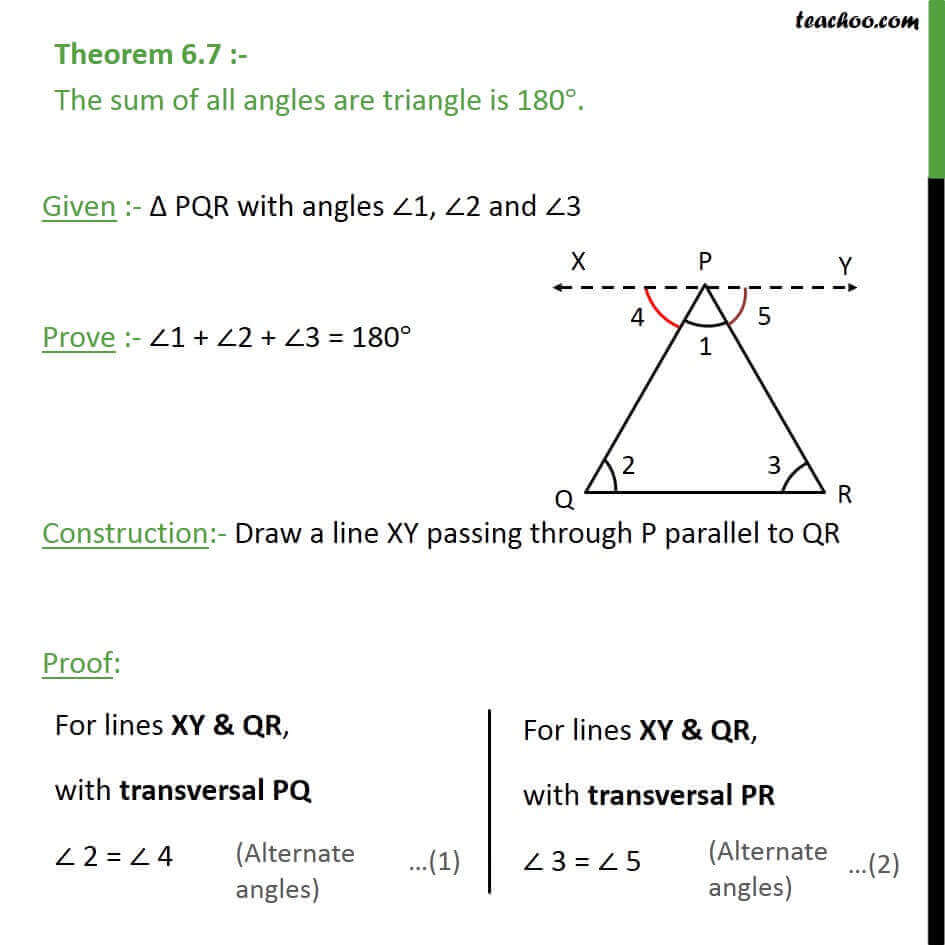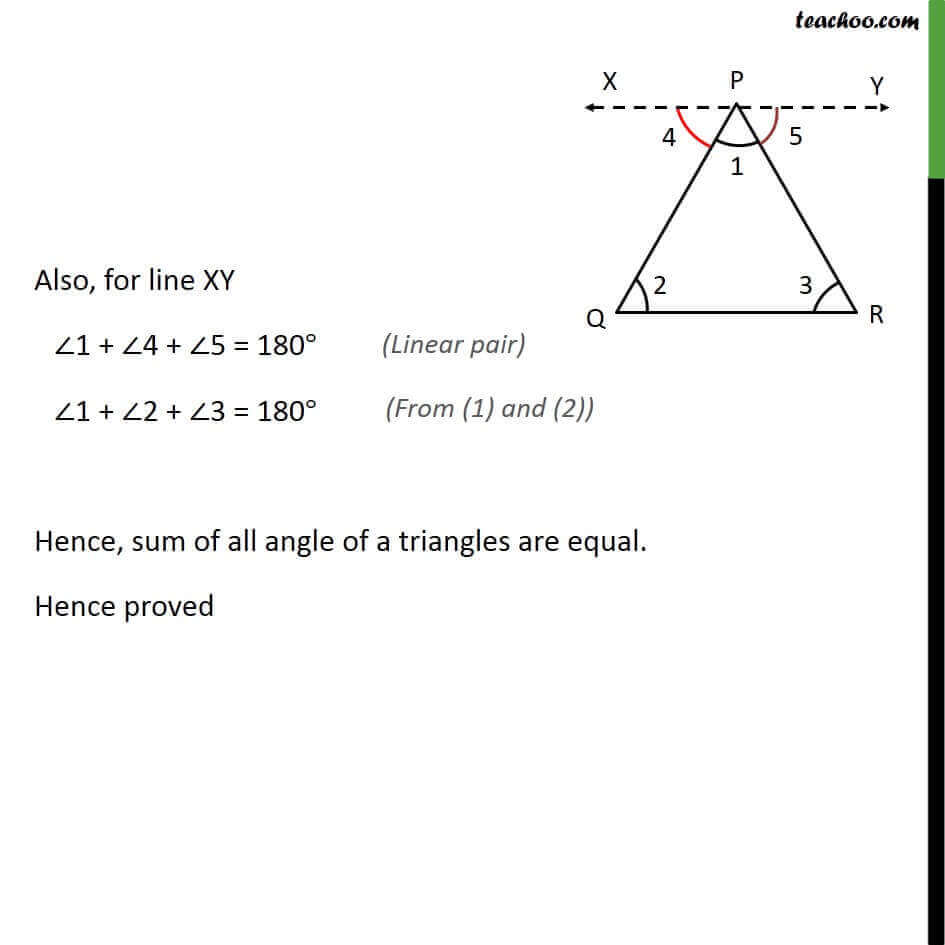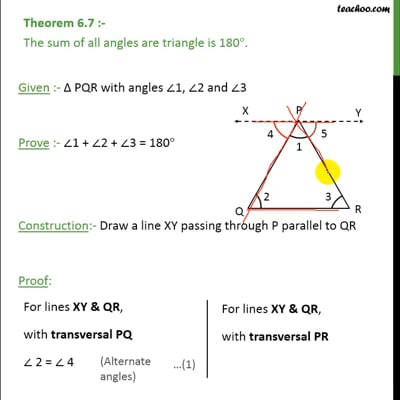Theorems

Chapter 6 Class 9 Lines and Angles
Serial order wiseThis video is only available for Teachoo black users

Get live Maths 1-on-1 Classs - Class 6 to 12

### Transcript

Theorem 6.7 :- The sum of all angles are triangle is 180°. Given :- Δ PQR with angles ∠1, ∠2 and ∠3 Prove :- ∠1 + ∠2 + ∠3 = 180° Construction:- Draw a line XY passing through P parallel to QR Proof: Also, for line XY ∠1 + ∠4 + ∠5 = 180° ∠1 + ∠2 + ∠3 = 180° Hence, sum of all angle of a triangles are equal. Hence proved